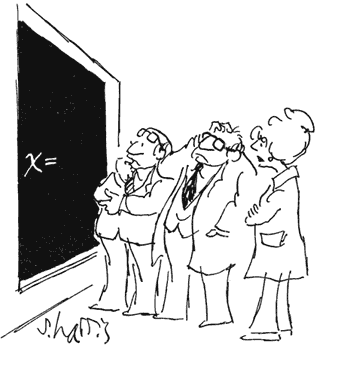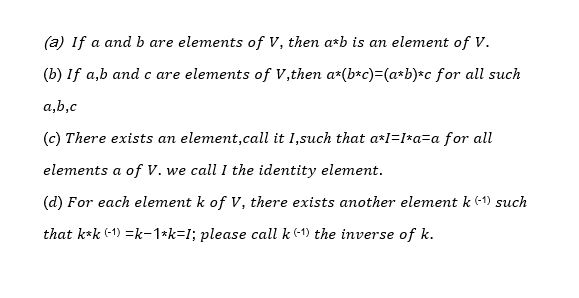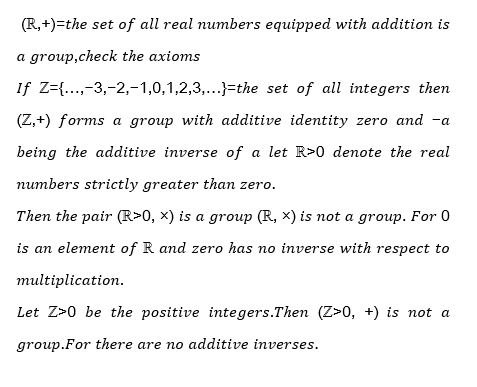# Briskly on Group TheoryThis is a ‘brisk’ mathematical introduction to Group Theory. In maths, we define things by listing a bunch of rules and anything that satisfies all these rules, fits the definition. We call these rules axioms. This article is a meander towards understanding some of the axioms that define a mathematical group. One of the goals of mathematics is to take things that are familiar to us and generalise them ? you might notice that a lot of the axioms defined are really similar to the familiar rules of addition and multiplication for numbers.

The topic of Geometric group theory was brought up by our head of college at our most recent formal dinner, it being the topic of the master’s thesis of one the tutors in this house( Tian Sang, congratulations!). Personally, I always have a warm smile( internally, at least) when Deborah mentions mathematical topics. I’d like to give Group Theory more exposure, with what little knowledge I have of this topic that must be so inspiring to its artisans.

Consider the concept of a set. An example of a set is as follows:A mathematician would call (†) ‘The set A, whose elements are 1,2,3,4 and 5’. So a set starts off with two key concepts: The container and its contents, the elements of the set. Elements can include numbers, matrices, functions and even other sets, but the main point is that elements are just ‘things’ that fill the set. Clearly, since 0 is a number, it would be interesting to point out that the following are not the same: { } ≠{0}

To some it is obvious, but to others it may be odd, as zero is often associated with ‘nothing’. It comes in the distinguishing fact that 0 is indeed a number and that in the mathematics of the theory of sets, nothing is not the same as the presence of the element zero, because zero is a something that has a precise meaning( I mean to say that it is an element to go in sets, just like any other).

The next step is to consider a binary operation. Everyone is familiar with the concept of a binary operation, because two such things are ubiquitous, addition and multiplication. Binary operations are rules which take two elements( hence binary) from a set and associate that pair with one element from that set. We then say that the binary operation is acting on the set. For example, the expression

1+2=3

uses the binary operation + to assign to the pair of numbers (1,2) the number 3

It turns out that there are many more examples of binary relations, other than multiplication and addition. Thus, let * denote a general binary which acts on a set. With this, we now have access to the following to knowing what a group is.

Suppose we have some set, call it V, and that we have a binary operation, *, which acts on that set. If we have two such things, let me denote (V,*) “the set V which has associated with it a binary operation * “.

(V,*) is a group if the following axioms are satisfied:And that is it. A group, in the mathematical sense, thus. If anyone ever asks you if you know any group theory, then proudly say yes!

Some examplesEver so hopefully, articles on mathematics to be continued.